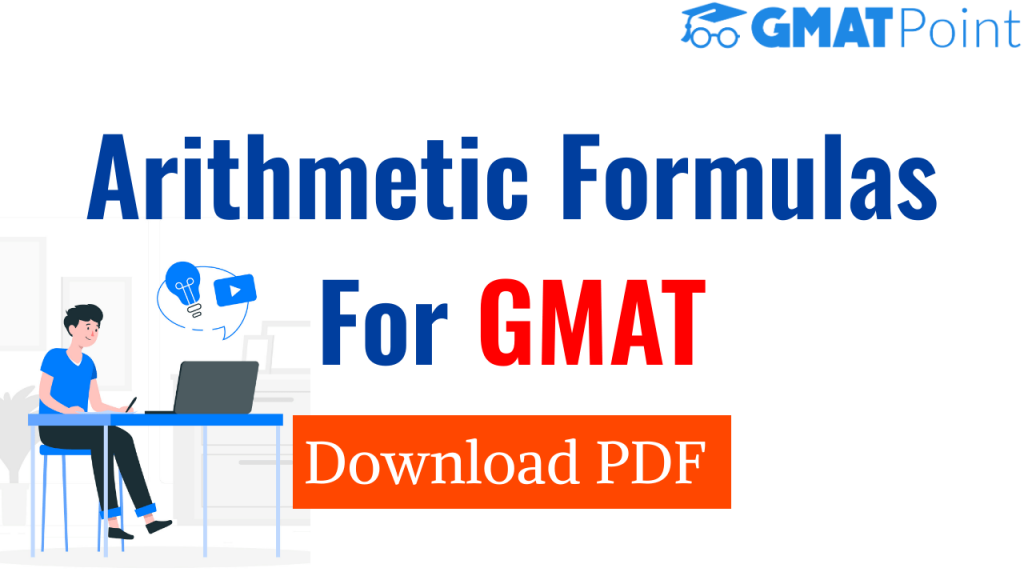# GMAT Arithmetic Formulas | Arithmetic Cheat Sheet [PDF]# GMAT Arithmetic Formulas | Arithmetic Cheat Sheet PDF

To achieve a good score on the Quantitative section, it is essential that you memorize the necessary GMAT Math Formulas. Below you will be finding PDF for Arithmetic complete formulas required for the GMAT Preparation. While preparing solving problems this PDF will be very useful. Befor that, one should be aware of the examination pattern clearly and have a proper GMAT preparation paln

Take Free GMAT Tests

## GMAT Arithmetic Formulas:

Before we get started, we’ll need to define a few terminology. A sequence, also known as a series, is a group of numbers that can be expressed in a certain order or can simply be a random set of numbers. Arithmetic sequences, also known as arithmetic formulas or arithmetic series, are a set of integers that follow a specified pattern. The pattern is determined by multiplying each number in the sequence by a specified quantity to get the next number in the sequence. The number added for each word in the sequence must be the same, and this number is known as the common difference.

These Arithmetic formulas are used in GMAT Quant Section. They can be used to determine patterns in architecture and also have uses in working with finances.

Various topics covered in this Arithmetic Formulas [PDF] are :

• Properties of Integers
• Fractions
• Decimals
• Real Numbers
• Ratio and Proportions
• Percentages
• Power and Roots of Numbers
• Descriptive Statistics Sets
• Counting Methods
• Discrete Probability
• Profit, Loss and Discounts

Arithmetic operations, such as add, subtract, multiply, and divide, are part of the basic Maths formulas. Algebraic identities also aid in the solution of equations. The following are some of the formulas:

It is necessary to know the first term of the sequence, the number of terms, and the common difference in order to calculate the formulas for an arithmetic sequence. Now,

Arithmetic  Formulas
nth Term Formula an = a1 + (n – 1)d
Sum of First n Terms S= n/2 (first term + last term)

Where, an = nth term that has to be found, a1 = 1st term in the sequence, n = Number of terms, d = Common difference, Sn = Sum of n terms

A series with a constant difference between terms, such as 1, 5, 9, 13, 17 or 12, 7, 2, –3, –8, –13, –18. The first term is referred to as a1, the common difference is referred to as d, and the total number of terms is n.

While some sequences consist solely of random values, others follow a specific pattern to arrive at the sequence’s terms.

You will learn the Arithmetic Sequence Explicit formula as well as a few solved examples to assist you comprehend this mathematical formula.

The formula for Arithmetic Sequence Explicit is as follows: an = a1 + (n – 1)d, i.e, an is the nth term in the sequence, a1 is the first term in the sequence, n is the term number, d is the common difference.

We have covered all the arthmetics topics of formulas in the PDF.

Check out the PDFs for Ratio & Proportion Formulas, Profit and Loss Formulas.